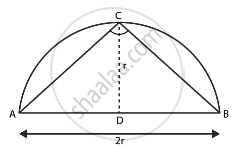# Area of the largest triangle that can be inscribed in a semi-circle of radius r units is ______. - Mathematics

MCQ

Area of the largest triangle that can be inscribed in a semi-circle of radius r units is ______.

#### Options

• rsq.units

• 1/2 rsq.units

•  2 rsq.units

• sqrt(2) rsq.units

#### Solution

Area of the largest triangle that can be inscribed in a semi-circle of radius r units is rsq.units.

Explanation:

The largest triangle that can be inscribed in a semi-circle of radius r units is the triangle having its base as the diameter of the semi-circle and the two other sides are taken by considering a point C on the circumference of the semi-circle and joining it by the endpoints of diameter A and B.∴ ∠ C = 90°  .....(By the properties of circle)

So, ΔABC is right-angled triangle with base as diameter AB of the circle and height be CD.

Height of the triangle = r

∴ Area of largest ΔABC = 1/2× Base × Height

= 1/2 × AB × CD

= 1/2 × 2r × r

= r2 sq.units

Concept: Area of Circle
Is there an error in this question or solution?

#### APPEARS IN

RD Sharma Class 10 Maths
Chapter 13 Areas Related to Circles
Exercise 13.6 | Q 49 | Page 74
NCERT Mathematics Exemplar Class 10
Chapter 11 Area Related To Circles
Exercise 11.1 | Q 4 | Page 121

Share